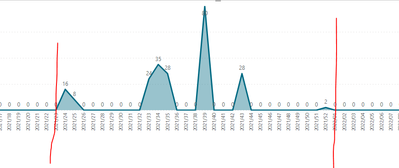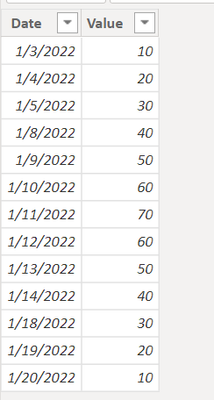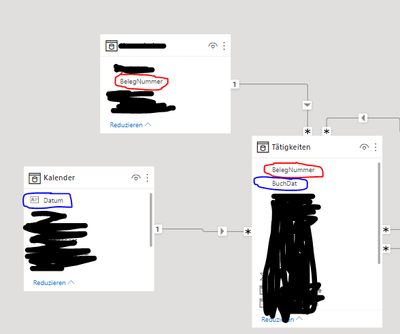cancel
Showing results for
Did you mean:Frequent Visitor

## Blank as Zero but only between first and last date which have a value greater 0

Hello together,

i am looking for a way to show blanks as zero, but only between the first and last date which have Values > 0.

Usually i do it with +0 but then i have the problem, that every value in calendar gets a 0.

i need something like a for loop:

for all dates which are > calculate(min(date), value > 0) && < calculate(max(date), value > 0),
value + 0,

value

Thanks for help!

Nico

6 REPLIES 6Super User

@Nico78 , Try like

0 between range
Measure = var _1= SUM(Opportunity[Opportunity count]) +0
var _min = minx(ALLSELECTED('Calendar'), 'Calendar'[Date])
var _max = maxx(ALLSELECTED('Calendar'), 'Calendar'[Date])
return
CALCULATE(if(max('Calendar'[Date]) <_min || max('Calendar'[Date]) >_max , BLANK(), _1))Frequent Visitor

doesn't get the expected solution.Community Support

Hi, @Nico78

Example data:Table:

``Date = CALENDAR(DATE(2022,1,1),DATE(2022,1,31))``

Measure:

``````Measure =
VAR N1 =
SUM ( 'Table'[Value] ) + 0
VAR Mindate =
CALCULATE ( MIN ( 'Table'[Date] ), ALLEXCEPT ( 'Table', 'Table'[Date] ) )
VAR Maxdate =
CALCULATE ( MAX ( 'Table'[Date] ), ALLEXCEPT ( 'Table', 'Table'[Date] ) )
RETURN
IF (
SELECTEDVALUE ( 'Date'[Date] ) >= Mindate
&& SELECTEDVALUE ( 'Date'[Date] ) <= Maxdate,
N1,
BLANK ()
)``````Is this the result you expect?

Best Regards,

Community Support Team _Charlotte

If this post helps, then please consider Accept it as the solution to help the other members find it more quickly.Frequent Visitor

I think its the right way to go, but i have one problem left. In your example there ist no dimension table (except date table) to filter the fact table. But i have another column in my fact table which i have to filter with another dimension table. What now happens is that only the dates from the fact table get a zero as blank (which is good for the moment) but the measure does not recognize the min and max date for a specific dimension filter. This means that the minimum date value in fact table always gets a zero even if the minimum date for a specific dimension filter is much later.

Do you know a solution for this problem?

many many thanks!

NicoCommunity Support

Hi, @Nico78

Can you provide a simple example file? Sensitive data can be provided for deletion. The desired result can also be shown with a picture.

Best Regards,

Community Support Team _Charlotte

If this post helps, then please consider Accept it as the solution to help the other members find it more quickly.Frequent Visitor

Data model:Result without a filter selection "Belegnummer" (as expected and correct):Result with a filter selection "Belegnummer":It starts at 31.12.2019 because thats the first date in table "Tätigkeiten". But with one filter selection in "Belegnummer" it should start at the first date, where a value is greater 0 for the specific "Belegnummer".

Hope it is understandable.

Thanks again

Nico